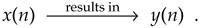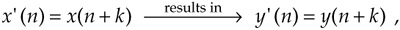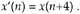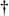# TIME-INVARIANT SYSTEMS

A time-invariant system is one where a time delay (or shift) in the input sequence causes a equivalent time delay in the system's output sequence. Keeping in mind that n is just an indexing variable we use to keep track of our input and output samples, let's say a system provides an output y(n) given an input of x(n), orFor a system to be time invariant, with a shifted version of the original x(n) input applied, x'(n), the following applies:

Equation 1-24where k is some integer representing k sample period time delays. For a system to be time invariant, expression (1-24) must hold true for any integer value of k and any input sequence.

1.6.1 Example of a Time-Invariant System

Let's look at a simple example of time invariance illustrated in Figure 1-9. Assume that our initial x(n) input is a unity-amplitude 1-Hz sinewave sequence with a y(n) output, as shown in Figure 1-9(b). Consider a different input sequence x'(n), where

Equation 1-25Figure 1-9. Time-invariant system input-to-output relationships: (a) system block diagram where y(n) = –x(n)/2; (b) system input and output with a 1-Hz sinewave applied; (c) system input and output when a 1-Hz sinewave, delayed by four samples, is applied. When x'(n) = x(n+4), then, y'(n) = y(n+4).Equation (1-25) tells us that the input sequence x'(n) is equal to sequence x(n) shifted four samples to the left, that is, x'(0) = x(4), x'(1) = x(5), x'(2) = x(6), and so on, as shown on the left of Figure 1-9(c). The discrete system is time invariant because the y'(n) output sequence is equal to the y(n) sequence shifted to the left by four samples, or y'(n) = y(n+4). We can see that y'(0) = y(4), y'(1) = y(5), y'(2) = y(6), and so on, as shown in Figure 1-9(c). For time-invariant systems, the y time shift is equal to the x time shift.

Some authors succumb to the urge to define a time-invariant system as one whose parameters do not change with time. That definition is incomplete and can get us in trouble if we're not careful. We'll just stick with the formal definition that a time-invariant system is one where a time shift in an input sequence results in an equal time shift in the output sequence. By the way, time-invariant systems in the literature are often called shift-invariant systems.[]

[] An example of a discrete process that's not time-invariant is the downsampling, or decimation, process described in Chapter 10.

URL http://proquest.safaribooksonline.com/0131089897/ch01lev1sec6AmazonPrev don't be afraid of buying books Next